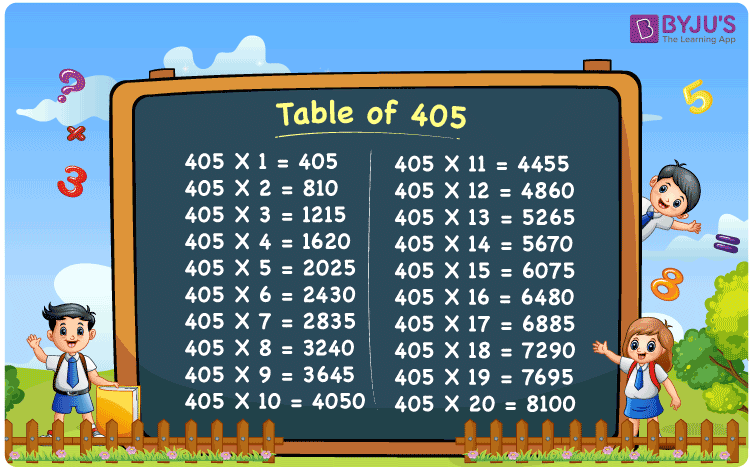Checkout JEE MAINS 2022 Question Paper Analysis : Checkout JEE MAINS 2022 Question Paper Analysis :

# Table of 405

The Table of 405 is the multiplication table of 405 that includes its multiples. 405 times table represents the continuous addition of number 405 to itself. It is vital for students to memorise the table of 405 comprehensively to solve complex problems with ease. Solving the problems with the help of multiplication tables increases students’ problem-solving capabilities.## What is the 405 Times Table?

The 405 times table is regarded as the multiplication table of number 405. Multiplication tables are essential in Mathematics. Students must learn the table of 405 to solve textbook problems effortlessly. Refer to the chart mentioned below to learn the table of 405 for the first ten times.

 405×1 = 405 405 405×2 = 810 405 + 405 = 810 405×3 = 1215 405 + 405 + 405 = 1215 405×4 = 1620 405 + 405 + 405 + 405 = 1620 405×5 = 2025 405 + 405 + 405 + 405 + 405 = 2025 405×6 = 2430 405 + 405 + 405 + 405 + 405 + 405 = 2430 405×7 = 2835 405 + 405 + 405 + 405 + 405 + 405 + 405 = 2835 405×8 = 3240 405 + 405 + 405 + 405 + 405 + 405 + 405 + 405 = 3240 405×9 = 3645 405 + 405 + 405 + 405 + 405 + 405 + 405 + 405 + 405 = 3645 405×10 = 4050 405 + 405 + 405 + 405 + 405 + 405 + 405 + 405 + 405 + 405 = 4050

## Multiplication Table of 405

Multiplication tables help students in solving complex multiplication problems quickly. Students can go through the table of 405 from 1 to 20 times given below.

 405 × 1 = 405 405 × 2 = 810 405 × 3 = 1215 405 × 4 = 1620 405 × 5 = 2025 405 × 6 = 2430 405 × 7 = 2835 405 × 8 = 3240 405 × 9 = 3645 405 × 10 = 4050 405 × 11 = 4455 405 × 12 = 4860 405 × 13 = 5265 405 × 14 = 5670 405 × 15 = 6075 405 × 16 = 6480 405 × 17 = 6885 405 × 18 = 7290 405 × 19 = 7695 405 × 20 = 8100

## Solved Example on the Table of 405

Example 1:

Solve 405 times 13 minus 405 times 9.

Solution:

405 times 13 = 405 x 13 = 5265

405 times 9 = 405 x 9 = 3645

405 times 13 minus 405 times 9 = 5265 – 3645 = 1620

## Frequently Asked Questions on Table of 405

### What is 405 times 19 plus 100 minus 50?

405 times 19 plus 100 minus 50
= 405 x 19 + 100 – 50
= 7695 + 100 – 50
= 7745

### What times of 405 is 4860?

12 times 405 is 4860.

### Using the table of 405, find 405 times 13 plus 90 minus 35.

405 times 13 plus 90 – 35
= 405 x 13 + 90 – 35
= 5265 + 90 – 35
= 5320